## MP Board Class 9th Maths Solutions Chapter 13 Surface Areas and Volumes Ex 13.7

Assume π = $$\frac{22}{7}$$, unless stated otherwise.Question 1.
Find the volume of the right circular cone with

1. radius 6 cm, height 7 cm
2. radius 3.5 cm, height 12 cm.

Solution:
1. Here, radius of the cone r = 6 cm
height (h) = 7 cm
Volume = $$\frac{1}{3}$$ x πr2h
= $$\frac{1}{3}$$ x $$\frac{22}{7}$$ x 6 x 6 x 7 cm3
= 22 x 2 x 6 cm3
= 264 cm3

2. Here, radius of the cone (r) ;
= 3.5 cm = $$\frac{35}{10}$$ cm
Height (h) = 12 m
Volume of the coneQuestion 2.
Find the capacity in litres of a conical vessel with

1. radius 7 cm, slant height 25 cm
2. height 12 cm, slant height 13 cm

Solution:
1. Here, r = 7 and l = 25 cm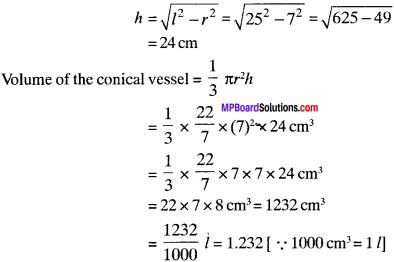Thus, the required capacity of the conical vessel is 1.232 l.

2. Here, height (h) – 12 cm and l = 13 cm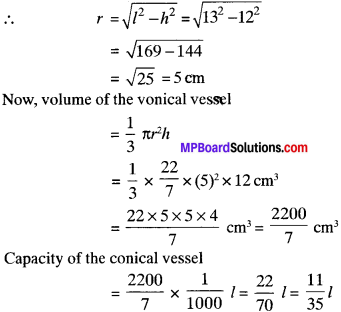Thus, the required capacity of the conical vessel is $$\frac{11}{35}$$ l.

Question 3.
The height of a cone is 15 cm. If its volume is 1570 cm3, find the radius of the base. (Use 71 = 3.14)
Solution:
Here, height of the cone (h) = 15 cm
Volume of the cone (v) = 1570 cm3
Let the radius of the base be ‘r’ cm.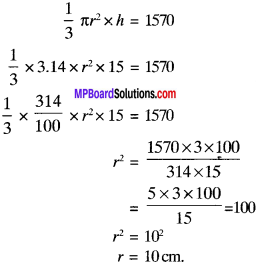Question 4.
If the volume of a right circular cone of height 9 cm is 48 JI cm3, find the diameter of its base.
Solution:
Volume of cone = $$\frac{1}{3}$$ πr2h
$$\frac{1}{3}$$ x πr2 x 9 = 48π
r2 = $$\frac{48π}{9π}$$ x 3
r2 = 16
r = 4 cm
Diameter = 2 x 4 = 8 cmQuestion 5.
A conical pit of top diameter 3,5 m is 12 m deep. What is its capacity in kilolitres?
Solution:
d = 3.5 m
r = 1.75 m
h = 12m
Volume of the pit = $$\frac{1}{3}$$ x $$\frac{22}{7}$$ x 1. 75 x 1.75 x 12
= 38.5 m3 = 38.5 kl (1 kl= 1 m3)

Question 6.
The volume of a right circular cone is 9856 cm3. If the diameter of the base 28 cm, find.

(i) height of the cone.
(ii) slant height of the cone.
(iii) curved surface area of the cone.

Solution:
V = 9856 cm2
d =28 cm
r = 14 cm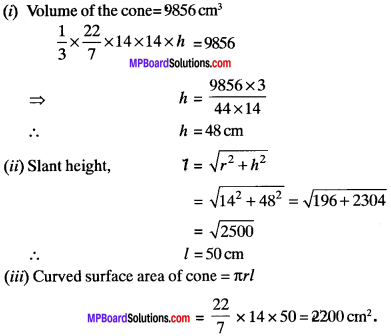Question 7.
A right triangle ABC with sides 5 cm, 12 cm and 13 cm is revolved about the sides 12 cm. Find the volume of the solid 30 obtained.
Solution:
h = 12cm
r = 5cm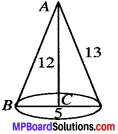Volume of solid, V1 = $$\frac{1}{3}$$πr2h
= $$\frac{1}{3}$$ x π x 5 x 5 x 12
= 100π cm3

Question 8.
If the triangle ABC in the Question 7 above is revolved about the side 5 cm, then find the volume of the solid so obtained. Find also the ratio of the volumes of the two solids obtained in Questions 7 and 8.
Solution:
h = 5 cm
r = 12 cm
Volume of solid, V2 = $$\frac{1}{3}$$ x π x 12 x 12 x 5
= 240π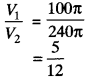Question 9.
A heap of wheat is in the form of a cone whose diameter is 10.5 m and height is 3 m. Find its volume. The heap is to be by covered canvas to protect it from rain. Find the area of the canvas required.
Solution:
d = 10.5 m ⇒ r = 5.25 m
h = 3m
Volume of heap of wheat = $$\frac{1}{3}$$ x $$\frac{22}{7}$$ x 5.25 x 5.25 x 3
= 86.625 m3
l2 = 32 + (5.25)2
l = $$\sqrt{9+27.56}$$ = $$\sqrt{36.56}$$ = 6.04 cm
Area of canvas required = CSA of cone –
= $$\frac{22}{7}$$ x 5.25 x 6.04 = 99.66 m2